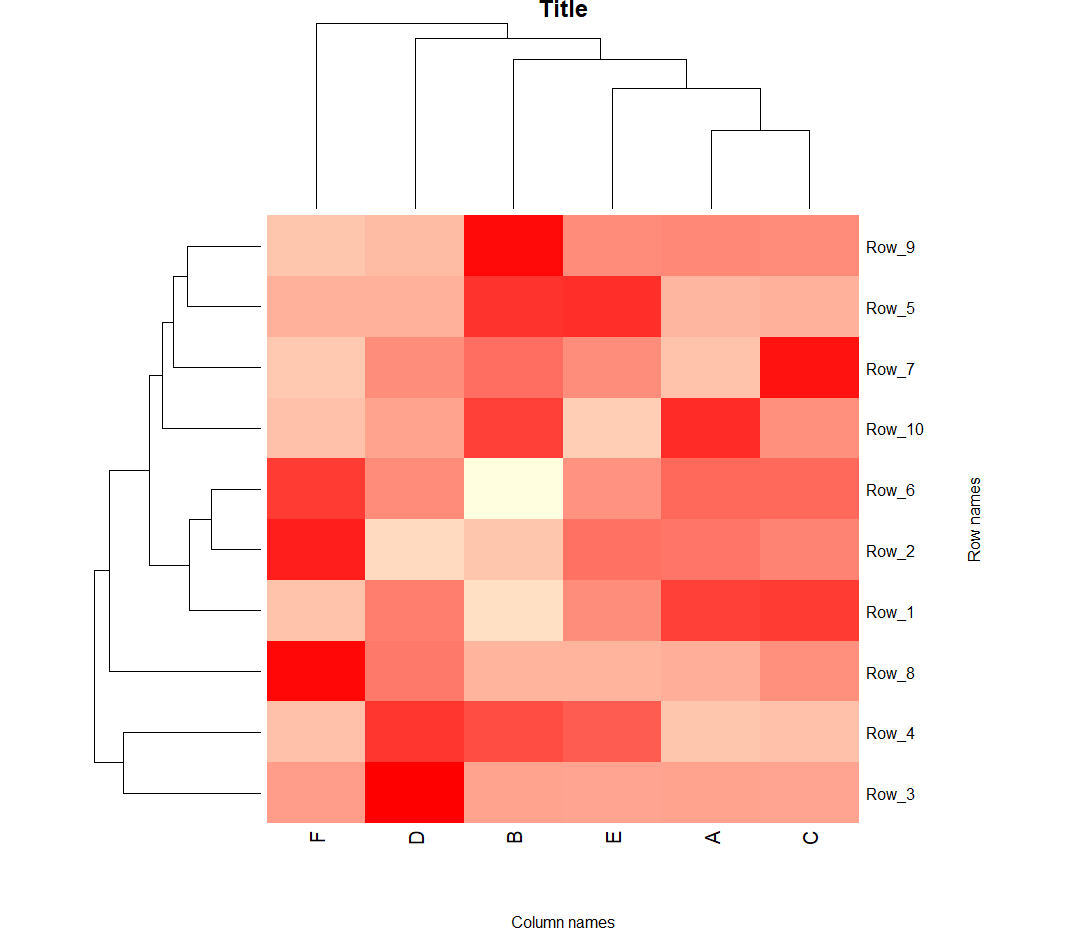## Clustering and Heatmap generation using R

Clustering and heatmap helps us to visualize trends in large dataset. Here, this method will describe how to create one in R

## Preparing the dataset

Ideal dataset for heatmap is a matrix (preferably as a csv file), where there are rows and columns of data, like this:

``````\$ cat test_dataset.txt
A	B	C	D	E	F
Row_1	97	14	99	65	57	29
Row_2	56	18	50	8	58	98
Row_3	58	58	43	936	47	78
Row_4	2	55	5	65	48	5
Row_5	45	87	47	47	88	47
Row_6	69	6	69	55	52	88
Row_7	8	32	58	23	23	7
Row_8	6	3	21	32	3	87
Row_9	37	98	36	12	36	7
Row_10	85	75	34	25	2	9
``````

This is a tab separated data, simply use the `sed` to make it csv file

``````sed 's/\t/,/g' test_dataset.txt > test_dataset.csv
``````

The new data file should look like this:

``````\$ cat test_dataset.csv
,A,B,C,D,E,F
Row_1,97,14,99,65,57,29
Row_2,56,18,50,8,58,98
Row_3,58,58,43,936,47,78
Row_4,2,55,5,65,48,5
Row_5,45,87,47,47,88,47
Row_6,69,6,69,55,52,88
Row_7,8,32,58,23,23,7
Row_8,6,3,21,32,3,87
Row_9,37,98,36,12,36,7
Row_10,85,75,34,25,2,9
``````

## Creating the heatmap

Open R in either Windows/Linux/Mac. You will need following packages for this tutorial. If you don’t have them, please install using the following commands:

``````install.packages(c("gplots", "vegan", "RColorBrewer", "Cairo"))
source("https://bioconductor.org/biocLite.R")
biocLite("Heatplus")
``````

Once done, proceed with the follwing commands to generate heatmap.

``````# load required packages
library(gplots)
library(Heatplus)
library(vegan)
library(RColorBrewer)
library(Cairo)
# import the data file as matrix
all.data <- as.matrix(read.csv ("test_dataset.csv", sep=",", row.names=1))
# take a look at your data
all.data[1:3, 1:4]
# convert the data into proportions, you can also log transform your data
data.prop <- all.data/rowSums(all.data)
# view transformed data
data.prop[1:3, 1:3]
# create a color gradient for heatmap
scaleyellowred <- colorRampPalette(c("lightyellow", "red"), space = "rgb")(100)
# generate a heatmap without clustering
heatmap(as.matrix(data.prop), Rowv = NA, Colv = NA, col = scaleyellowred, margins = c(10, 2))
# Cluster the data, first rows
data.dist <- vegdist(data.prop, method = "bray")
row.clus <- hclust(data.dist, "aver")
# then columns, you can skip one of the clustering if you don't want to mess a particular row/column
data.dist.g <- vegdist(t(data.prop), method = "bray")
col.clus <- hclust(data.dist.g, "aver")
# generate a heatmap
p <- heatmap(as.matrix(data.prop),
Rowv = as.dendrogram(row.clus),
Colv = as.dendrogram(col.clus),
col = scaleyellowred,
margins = c(7, 8),
xlab = "Column names",
ylab = "Row names",
main = "Title", pch=10)
p
# to save it as scalable vector graphics (change svg to png, pdf etc if you need other formats)
svg("heatmap.svg")
p
dev.off()
``````

An example for how your heatmap will look is shown below:You can customize the axis titles, chart title by modifying the commands above.